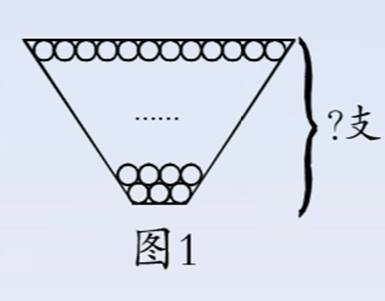## 如何巧妙配对求和

[问题1]

1.计算101+102+103+104+105+106+107+108+109

2.计算10+8+6+4+2-9+7+5+3+1

[思路点睛]

1题按照上一讲介绍的首尾配对或者颠倒配对求和计算量较大，但如果把每个数中的100分离出来，先算出9100，再算1+2+3+4+5+6+7+8+9，这样就简单多了。

2题可以先把加和减分别计算，但仔细观察发现，被减数里的5个加数与减数中的5个加数可以依次配对，分成5组，它们的差都是1，这样结果正好是5101+102+103+104+105+106+107+108+109=100×9+1+9）×9÷2=900+45=945。（10+8+6+4+2-9+7+5+3+1=10-9+8-7+6-5+4-3+2-1=1×5=5

?

[问题2]

[思路点睛]画出示意图分析（如图1），要求盒子里一共多少支，就是求3+4+5+6++11+12的和。利用首尾配对的方法可以很容易得出答案。3+4+5+6++11+12=3+12）×10÷2=15×5=75（支）。答：这个盒子里一共放有75支铅笔。[问题3]

[思路点睛]因为每个选手都要和其他选手各比赛一场，所以第一个人需要和其余19个人各比赛一场，第二个人因为已经和第一个人比赛过，只需要和剩下的18个人各比赛一场，这样依次类推，第三个人只需要再比赛17场，第四个人再比赛16场……因此问一共要进行多少场比赛，也就是求19+18+17++3+2+1的和。当然这道题的解题方法不止一种，大家可以进一步思考。